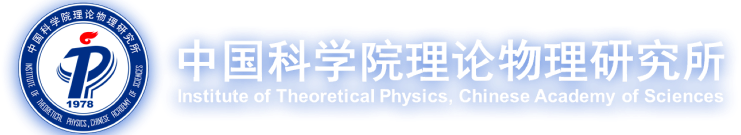#### 学术活动

05/28 2021 Seminar
• Title题目 Light $U(1)_{B-L}$ model at the high-intensity frontier
• Speaker报告人 Yongchao Zhang [Southeast University]
• Date日期 3:00 pm, May 28, 2021
• Venue地点 6420
• Abstract摘要
The low-energy $U(1)_{B-L}$ model is a well-motivated framework to account for the tiny neutrino masses. The light scalar ($\varphi$) and gauge ($Z’$) bosons in this model can be searched for in some high-intensity experiments. At the DUNE experiment, the gauge coupling of $\varphi$ to $Z’$ could improve significantly the $Z’$ prospects, even by one order of magnitude. The $Z’$ can also induce important interference effect for $\nu - e$ scattering at DUNE. Through mixing with the SM $Z$ boson, the light $Z’$ boson contributes to the low-energy high-precision P2 experiment, which is designed to measure the weak mixing angle in $e+p$ or $e + ^{12}$C scattering. If sufficiently light, these light particles in the $U(1)_{B-L}$ models can also be produced abundantly in the supernova cores and neutron star mergers, and the corresponding astrophysical observations could provide some constraints on the couplings of these light particles.

contact person: Jiang-Hao Yu